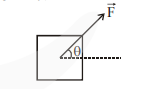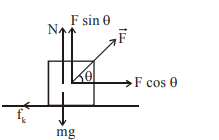# A block of mass m slides along a floor while a force of magnitude F is applied to it at an angle T as shown in figure.`
Question:

. A block of mass m slides along a floor while a force of magnitude F is applied to it at an angle T as shown in figure. The coefficient of kinetic friction is µK. Then, the block's acceleration 'a' is given by : (g is acceleration due to gravity)1. $-\frac{F}{m} \cos \theta-\mu_{K}\left(g-\frac{F}{m} \sin \theta\right)$

2. $\frac{\mathrm{F}}{\mathrm{m}} \cos \theta-\mu_{\mathrm{K}}\left(\mathrm{g}-\frac{\mathrm{F}}{\mathrm{m}} \sin \theta\right)$

3. $\frac{F}{m} \cos \theta-\mu_{K}\left(g+\frac{F}{m} \sin \theta\right)$

4. $\frac{F}{m} \cos \theta+\mu_{K}\left(g-\frac{F}{m} \sin \theta\right)$

Correct Option: 2,

Solution:$\mathrm{N}=\mathrm{mg}-\mathrm{f} \sin \theta$

$\mathrm{F} \cos \theta-\mu_{\mathrm{k}} \mathrm{N}=\mathrm{ma}$

$F \cos \theta-\mu_{k}(m g-F \sin \theta)=m a$

$a=\frac{F}{m} \cos \theta-\mu_{K}\left(g-\frac{F}{m} \sin \theta\right)$